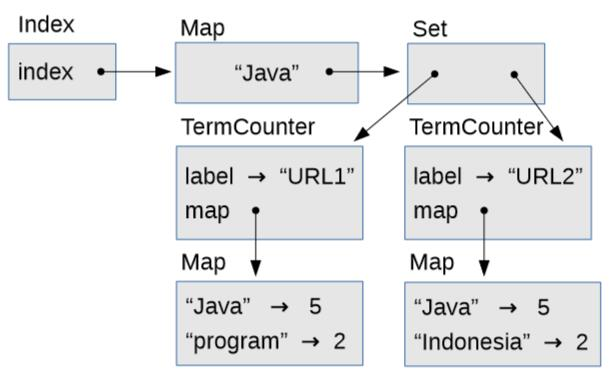# 第八章 索引器

## 8.1 数据结构选取

Java 提供了`Map`的调用接口，它指定映射应该提供的方法；最重要的是：

• `get(key)`：此方法查找一个键并返回相应的值。
• `put(key, value)`：该方法向`Map`添加一个新的键值对，或者如果该键已经在映射中，它将替换与`key`关联的值。

Java 提供了几个`Map`实现，包括我们将关注的两个，`HashMap`以及`TreeMap`。在即将到来的章节中，我们将介绍这些实现并分析其性能。

• `add(element)`：该方法将一个元素添加到集合中；如果元素已经在集合中，则它不起作用。
• `contains(element)`：该方法检查给定元素是否在集合中。

Java 提供了几个`Set`实现，包括`HashSet``TreeSet`

## 8.2 `TermCounter`

`TermCounter`是一个类，表示检索词到页面中出现次数的映射。这是类定义的第一部分：

``````public class TermCounter {

private Map<String, Integer> map;
private String label;

public TermCounter(String label) {
this.label = label;
this.map = new HashMap<String, Integer>();
}
}
``````

`TermCounter`提供`put``get`，定义如下：

``````    public void put(String term, int count) {
map.put(term, count);
}

public Integer get(String term) {
Integer count = map.get(term);
return count == null ? 0 : count;
}
``````

`put`只是一个包装方法；当你调用`TermCounter``put`时，它会调用内嵌映射的`put`

``````    public void incrementTermCount(String term) {
put(term, get(term) + 1);
}
``````

``````    public void processElements(Elements paragraphs) {
for (Node node: paragraphs) {
processTree(node);
}
}

public void processTree(Node root) {
for (Node node: new WikiNodeIterable(root)) {
if (node instanceof TextNode) {
processText(((TextNode) node).text());
}
}
}

public void processText(String text) {
String[] array = text.replaceAll("\\pP", " ").
toLowerCase().
split("\\s+");

for (int i=0; i<array.length; i++) {
String term = array[i];
incrementTermCount(term);
}
}
``````

``````    String url = "http://en.wikipedia.org/wiki/Java_(programming_language)";
WikiFetcher wf = new WikiFetcher();
Elements paragraphs = wf.fetchWikipedia(url);

TermCounter counter = new TermCounter(url);
counter.processElements(paragraphs);
counter.printCounts();
``````

## 8.3 练习 6

• `TermCounter.java`包含上一节中的代码。
• `TermCounterTest.java`包含测试代码`TermCounter.java`
• `Index.java`包含本练习下一部分的类定义。
• `WikiFetcher.java`包含我们在上一个练习中使用的，用于下载和解析网页的类。
• `WikiNodeIterable.java`包含我们用于遍历 DOM 树中的节点的类。

``````genericservlet, 2
configurations, 1
claimed, 1
servletresponse, 2
occur, 2
Total of all counts = -1
``````

``````public class Index {

private Map<String, Set<TermCounter>> index =
new HashMap<String, Set<TermCounter>>();

public void add(String term, TermCounter tc) {
Set<TermCounter> set = get(term);

// if we're seeing a term for the first time, make a new Set
if (set == null) {
set = new HashSet<TermCounter>();
index.put(term, set);
}
// otherwise we can modify an existing Set
}

public Set<TermCounter> get(String term) {
return index.get(term);
}
``````

`add`方法向集合添加新的`TermCounter`，它与检索词关联。当我们索引一个尚未出现的检索词时，我们必须创建一个新的集合。否则我们可以添加一个新的元素到一个现有的集合。在这种情况下，`set.add`修改位于`index`里面的集合，但不会修改`index`本身。我们唯一修改`index`的时候是添加一个新的检索词。`printIndex`方法展示了如何解压缩此数据结构：

``````    public void printIndex() {
// loop through the search terms
for (String term: keySet()) {
System.out.println(term);

// for each term, print pages where it appears and frequencies
Set<TermCounter> tcs = get(term);
for (TermCounter tc: tcs) {
Integer count = tc.get(term);
System.out.println("    " + tc.getLabel() + " " + count);
}
}
}
``````

``````public void indexPage(String url, Elements paragraphs) {
// 生成一个 TermCounter 并统计段落中的检索词

// 对于 TermCounter 中的每个检索词，将 TermCounter 添加到索引
}
``````

``````...
configurations
http://en.wikipedia.org/wiki/Programming_language 1
http://en.wikipedia.org/wiki/Java_(programming_language) 1
claimed
http://en.wikipedia.org/wiki/Java_(programming_language) 1
servletresponse
http://en.wikipedia.org/wiki/Java_(programming_language) 2
occur
http://en.wikipedia.org/wiki/Java_(programming_language) 2
``````